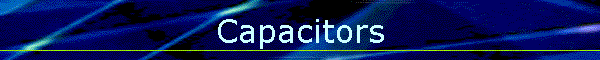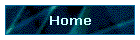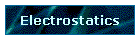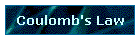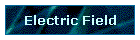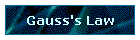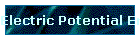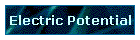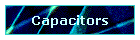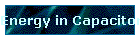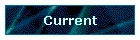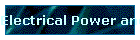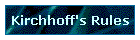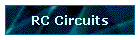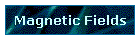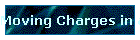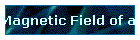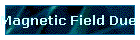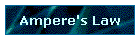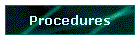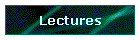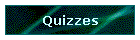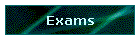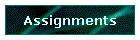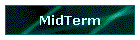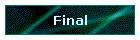Phys 4, Section 2                                                                 Jan 30, 2002 Capacitors A capacitor is a device composed of two conductive plates separated by an insulator. A capacitor is charged by moving charge from one plate to the other. Thus the charge on the two plates is equal and opposite, with the total charge on the device being zero. Its capacitance is defined at the ratio of the voltage across the two conductors to the charge on the positive plate. C = Q/V (units of farads) Actually, a farad is a very large unit, so in practice, microfarads and nanofarads, even picofarads, are more common units. The capacitance of a capacitor depends only on its geometry. The classic capacitor is assumed to be two large flat plates of area A separated by a distance d which is very small compared to the area. The space between the plates is filled with air or vacuum. In fact, many capacitors have insulating material, a dielectric, between the plates. This increases the capacitance. In the course, we will not use dielectric materials.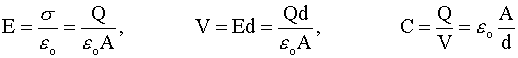Note that the capacitance is measured by assuming some test charge configuration, e.g., +Q on one plate, -Q on the other. However, the capacitance is a geometric quantity and has the same value even when the capacitor is uncharged. Capacitor Combinations Series: 1/Ceff = 1/C1 + 1/C2 + … Parallel: Ceff = C1 + C2 + …   Last modified on February 11, 2002